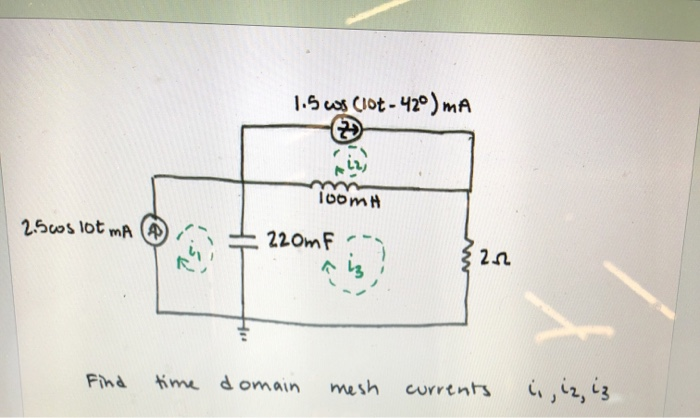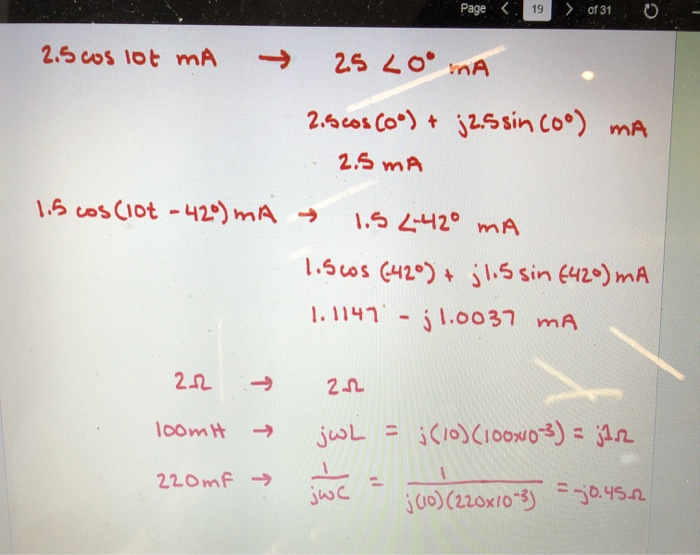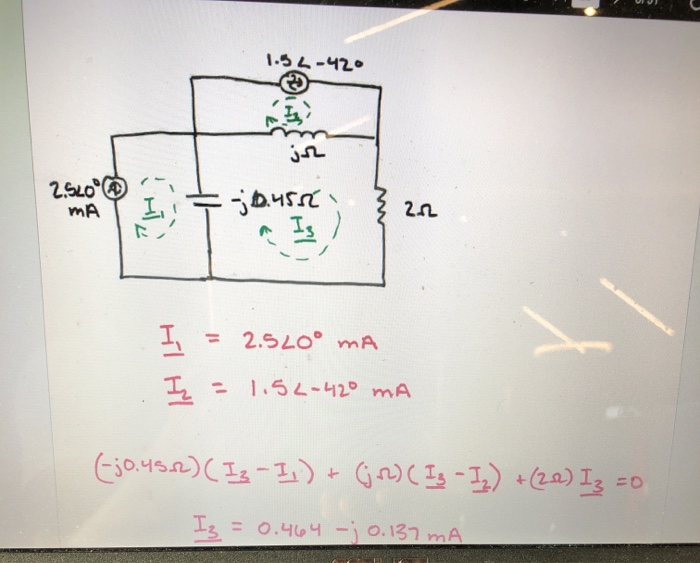# In the third photo can you show me the arithmitic to get I3 1.5 cos CIot-...

###### Question:

in the third photo can you show me the arithmitic to get I31.5 cos CIot- 42° ) mA ioomH 25os lot mA (A 220mF time domain mesh Find currents
Page < of 31 19 2.5 cos 1ot mA 25 LO mA -> 2.6 cos Co) + j2s sin co°) mA 2.5 mA 1.5 cos (Iot -42°) mA → 1.5 442° mA 1.5cos (42°) + jl.ssin 6420) mA j 1.0037 mA 1.1147 -> jwL = j (10) (ioowo3) = 12 1oomH -> 220mf > %3D jwc ju0) (220x/0 3)jo.45.2
1.54-420 In 2고 Is ) I, = 2.5LO° mA %3D In - 1.54-니20 mA %3D Ejo.4s2) ( Is-I,)+ Gr) ( Ig -Is) +(20) Iz =0 I3 = 0.464 -jo.137 mA

#### Similar Solved Questions

##### Suppose that the 64-bit string A B C D E F 9 8 7 6 5...
Suppose that the 64-bit string A B C D E F 9 8 7 6 5 4 3 2 1 0 is obtained after the initial permutation in DES. Compute the 32-bit strings L1 and R1 knowing that the round key K1 is 0 1 2 3 4 5 6 7 8 9 A B....
##### 3. Using no date-specific Java libraries, write a program that computes the number of days between...
3. Using no date-specific Java libraries, write a program that computes the number of days between any two dates (inclusive). You must take into account leap years. The rule for computing a leap year is as follows: If the year is divisible by 4 it is a Leap Year ... Unless the year is divisible by 1...